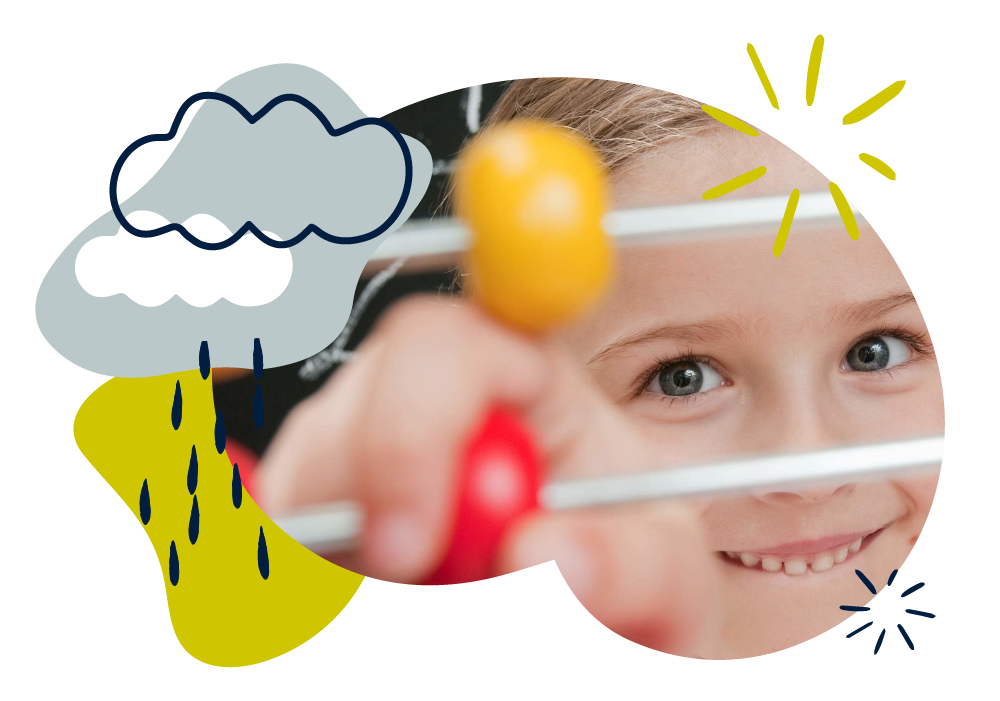We use cookies to enhance your experience on our website. By continuing to use our website, you are agreeing to our use of cookies. You can change your cookie settings at any time. Find out more# Maths: Age 5–6 (Year 1)

In Year 1, your child will start to build confidence working with numbers, through developing their counting and calculation skills. They will also gain an understanding of halves and quarters, start to measure and tell the time, and learn about some 2D and 3D shapes.

Your child will be taught to count forwards and backwards to 100, add and subtract numbers to 20, and be introduced to the idea of multiplying and dividing. They will be encouraged to use objects to help them solve simple problems in a practical way.

Much of your child’s learning will come from exploring and talking about maths in the world around them and there are simple things you can do at home to support their development.

## How to help at home

You don’t need to be an expert to support your child with maths or help them develop a good sense of number! Here are three simple, but effective learning ideas that you can try with your child using everyday items at home.

### 1. Count objects around the house

When counting, encourage your child to point to each object, putting them in a row. For more than 10 objects, group into tens to see that, for example, 32 is 3 tens and 2 ones. Practise counting in twos, fives or tens using, for example, pairs of socks, fingers on hands or 10p coins.

### 2. Play dice games

Gather some objects – blocks, buttons, even biscuits! Roll two dice and find the total, using the objects to add practically. Or start with, say, 12 objects, roll a dice and subtract the number shown on the dice to find how many objects are left. The player with more objects wins.

### 3. Use toys

Explore fractions using some of your child’s favourite toys, for example teddies or cars. Ask your child to halve their toys by splitting them into two equal groups. So, for ten cars, make two groups of five. Similarly, practise finding one quarter by splitting toys into four equal groups.

You’ll find more activity ideas in our Kids’ activities section.

## What your child will learn at school

#### Number & place value in Year 1 (age 5–6)

In Year 1, children will be expected to work with numbers up to 100, counting on or back from any number and in steps of 2, 5 or 10. This includes:

• reading and writing numerals to 100 and number names to 20 in words
• using objects and number lines to represent numbers
• finding one more and one less than any number.

#### Addition & subtraction in Year 1 (age 5–6)

In Year 1, children will be expected to be able to read, write and understand mathematical ideas using addition (+), subtraction (–) and equals (=) signs. This includes:

• making and using number bonds to 10 and then to 20
• adding and subtracting one-digit and two-digit numbers to 20, including 0
• solving simple problems using objects, drawings, diagrams and symbols, including missing number problems such as 7 = ? – 9.

#### Multiplication & division in Year 1 (age 5–6)

In Year 1, children will be expected to be able to solve simple multiplication and division problems using objects, drawings and arrays to help them. This includes:

• counting in steps of 2, 5 and 10 and understanding that, for example 3 × 2 is the same as 2 + 2 + 2
• using sharing and grouping to solve division problems
• beginning to understand the relationship between multiplication and division.

#### Fractions in Year 1 (age 5–6)

In Year 1, children will be expected to be recognise, find and name halves and quarters of objects, shapes and quantities. This includes:

• understanding halving as sharing equally into two parts or groups
• understand finding a quarter as sharing into four equal parts or groups
• understanding that finding a half is the same as dividing by 2 and finding a quarter is the same as dividing by 4.

#### Geometry in Year 1 (age 5–6)

In Year 1, children will be expected to be able to identify simple flat (2D) and solid (3D) shapes and use mathematical vocabulary to describe position, direction and movement. This includes:

• recognising and name common 2D shapes, such as circles, triangles, rectangles and squares
• recognising and name common 3D shapes, such as spheres, pyramids, cones, cuboids and cubes
• describing whole, half, quarter and three-quarter turns.